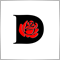# Experts: RSI Bollinger Bands EA - page 21031

I think I am getting it. So what period do you use for the Bollinger of the RSI? Standard 20 periods of the RSI? And is RSI set to the default 14 period? And the bands are set at what period deviations? Like 1.5 and 2.0? So in all 3 time frames we need to see the RSI bands in the zone between the 2 deviations and same with stochastics? Do you use the Bollinger on the Stochastic too or no need? And how do you come up with the stop loss and take profit parameters?

Thanks.5000

ForexTrader17:

I think I am getting it. So what period do you use for the Bollinger of the RSI? Standard 20 periods of the RSI? And is RSI set to the default 14 period? And the bands are set at what period deviations? Like 1.5 and 2.0? So in all 3 time frames we need to see the RSI bands in the zone between the 2 deviations and same with stochastics? Do you use the Bollinger on the Stochastic too or no need? And how do you come up with the stop loss and take profit parameters?

Thanks.

1. I use 60 periods or samples for determination of the Main and Upper/Lower bands of the RSI (external NumRSI).  This RSI itself uses a period of 20 (external RSIPer).  For other currencies I've used 10 and 14. The deviations come from the external data (RSIM15_Sigma_2,  RSIH1_Sigma_2, RSIH4_Sigma_2).  The deviations for the Upper/Lower Limit bands also come from the external data (RSIM15_SigmaLim_2,....).  I use additional  filters such as actual Bollinger Band spread ATR Limit and Stochastics.  I could have used the Bollinger Band concept for the Stochastic test also, but chose not  to because this is a secondary filter and and the computation costs additional processing time.  I may try to write an EA that replaces RSI with Stochastics for OB and OS zones.  I believe RSI works better.  The Stop Loss and Take Profit parameters are external data that are determined, along with the other external data, through the MT4 Tester Optimizer.  A Trailing Stop, not in this EA, also helps performance.   Also note that the T/P, S/L values for Long and Short trades are much different.

Thanks1031

What is Bollinger Band Spread ATR Limit used for? The other concept is clear. Use a say 20 period RSI with its 60 period Bollinger Bands and 2 deviations specified that will create a band around the RSI. If RSI goes in any it is OB/OS and must be sme in other time frames too. Can you add a Trailing Stop in there?

Thanks.1031

What is Bollinger Band Spread ATR anyways? The Higher Time Frames use the same 20 period RSI and same deviations off a 60 period Bollinger Band?

Thanks.5000

ForexTrader17:

What is Bollinger Band Spread ATR anyways? The Higher Time Frames use the same 20 period RSI and same deviations off a 60 period Bollinger Band?

Thanks.

Bollinger Band Spread  [Upper-Lower]/point and the ATR (volatility indicator) are used as additional filters of the data.  When the volatility is extremely high (say around the end of 2008) the performance is degraded, so this data has been filtered out.  Yes, the all time frames use the same RSI period, 20 in this case.  They also all use 60 samples in the respective time frames to compute the Stnd Deviation and Mean of the RSI distribution.   Of course, different factors are applied to the Stnd Deviation to compute the RSI band limits in each time frame.1031

Thanks. How often do you re-optimize? Isn't this kind of curve fitting? Do you need to optimize each pair? Can you explain how you use the Bollinger Spread? Is it like spread between upper and lower bands? Like all 4 or any 2? And what is used to calculate the ATR?

Thanks.

Thank you.5000

ForexTrader17:

Thanks. How often do you re-optimize? Isn't this kind of curve fitting? Do you need to optimize each pair? Can you explain how you use the Bollinger Spread? Is it like spread between upper and lower bands? Like all 4 or any 2? And what is used to calculate the ATR?

Thanks.

Thank you.

Every currency pair must be optimized separately. Also, optimization differs for each history database source (FXDD, Metaquotes, FXCM...).  I use an upper limit for the BB Spread ((upper-lower)/point) in the M15 time frame and a minimum BB spread in the H4 time frame.   The ATR is taken from the ATR indicator.  The H4 ATR and M15 BB Spread max limits remove high volatility periods of time.  These setting and corresponding periods and limits all can be seen in the External data.

Thanks1031

Thanks. So if there is a nin. and max. BB Spread how do you work around that in optimizing?

Thanks.10611

Looks promising5000

ForexTrader17:

Thanks. So if there is a nin. and max. BB Spread how do you work around that in optimizing?

Thanks.

I use BBSpread  Minimum in the H4 time frame and BBSpread Max in the M15 time frame.  These are separate externals that can be independently optimized.